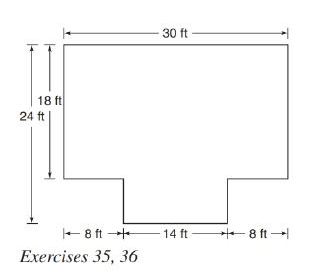Chapter 8.2, Problem 35EElementary Geometry For College St...

7th Edition
Alexander + 2 others
ISBN: 9781337614085

Solutions

Chapter
SectionElementary Geometry For College St...

7th Edition
Alexander + 2 others
ISBN: 9781337614085
Textbook Problem

Find the area of the room whose floor plan is shown.To determine

To Find:

Area of the room provided the floor plan.

Explanation

Formula Used:

Split the octagon into two rectangles and find the corresponding areas and then sum it up.

Area of rectangle =b×h

Calculation:

Let the vertices of the provided octagon be A, B,C, D, E, F, G and H.

Let’s divide the octagon into two rectangles. Draw a line CF the point C to F.

Here ABGH and CDEF both forms rectangles.

It is given that AB=18 ft, BC=FG=8 ft, DE=14 ft, and AH=30 ft.

Since the total height of the octagon is 24 ft and AB=18 ft, CD must be 24-18=6 ft

Still sussing out bartleby?

Check out a sample textbook solution.

See a sample solution

The Solution to Your Study Problems

Bartleby provides explanations to thousands of textbook problems written by our experts, many with advanced degrees!

Get Started

Find f. f(x) = 20x3 12x2 + 6x

Single Variable Calculus: Early Transcendentals, Volume I

Solve the equations in Exercises 126. x+4x+1+x+43x=0

Finite Mathematics and Applied Calculus (MindTap Course List)

In Exercises 73-80, find the indicated limits, if they exist. 80. limx2x21x3+x2+1

Applied Calculus for the Managerial, Life, and Social Sciences: A Brief Approach

Find the mean for the scores in the following frequency distribution table: X f 6 1 5 4 4 2 3 2 2 1

Essentials of Statistics for The Behavioral Sciences (MindTap Course List)

0 1 does not exist

Study Guide for Stewart's Single Variable Calculus: Early Transcendentals, 8th